## 4.2 Dominance Constraints

We now present a formal language with which we can write tree descriptions. This is the language of Dominance Constraints with Set Operators as described in [DN00]. It has the following abstract syntax: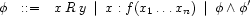where variables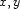denote nodes and.represents proper dominance and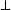disjointness. The constraintis satisfied when the relationship that holds betweenandis one in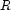. Thus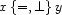is satisfied either whenandare equal, or when they denote nodes in disjoint subtrees. We write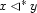instead of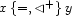and generally omit braces when we can write a single symbol.

Consider again the scope ambiguity example: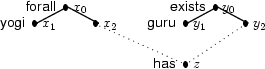It can be expressed as the following dominance constraint: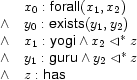Denys Duchier
Version 1.2.0 (20010221)